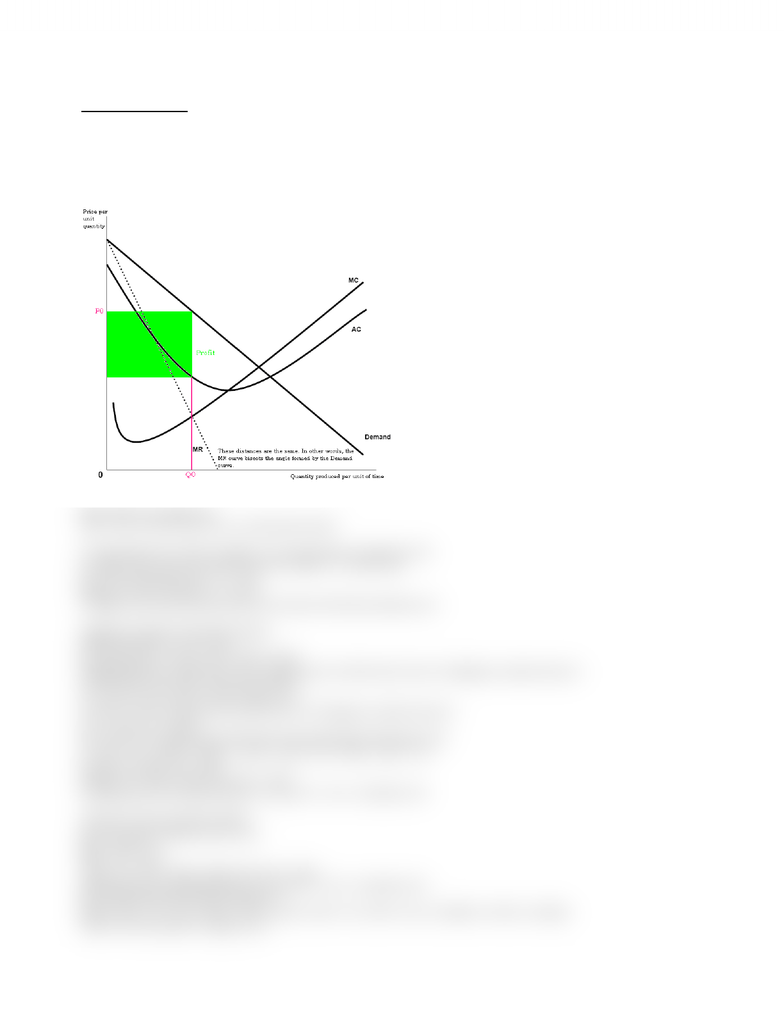# Lecture notes week 8

66 views2 pagesECMA04H – Week Eight
The Monopoly Model
a. The monopoly model. How does monopolist behave? What are the results?
b. Inefficiency and monopoly. Dynamic efficiency vs. allocative efficiency.
c. Where does monopoly come from; what conditions are necessary; what are the public policy responses?
d. Excise tax on a monopoly
i Only one supplier. Entry to market is blocked.
i Single seller faces entire market demand curve (price maker, not price taker)
MR = dTR / dq or dTR / dQ
TR = P x Q. To PC firm, P was a constant (price taker)
To monopolist, P is a choice variable, so we must treat P as a function of Q
So, MR = [(dP / dQ) x Q] + [(dQ / dQ) x P] or MR = P + Q (dP / dQ)
For a linear demand curve P = a – b Q,
MR = (a – b Q) + (Q [-b]) = a – 2 b Q
So MR has the same intercept and twice the slope of the linear demand curve
Algebraic example of monopoly industry
Market Demand: P = 100 - .02Q
So, total revenue = TR = P x Q = 100Q - .02Q2
Marginal Revenue = dTR / dQ = 100 - .04Q (the rate at which total revenue is changing as output increases)
Assume total cost function of monopoly firm is:
TC = .01q2 + 10q + 432 or .01Q2 + 10Q + 432
So, MC = .02Q + 10 (the rate at which total cost is changing as output increases)
AC = .01Q + 10 + 432Q-1
We can solve by forming the profit function and maximizing with respect to Q
3 = TR – TC = (100Q - .02Q2) – (.01Q2 + 10Q + 432) = 90Q - .03Q2 – 432
Therefore, d3/dQ = 90 - .06Q
Setting = 0, we have .06Q = 90 or Q* = 1500
Substituting into the demand function, we have P* = 100 - .02(1500)= \$70
Alternative (easier) solution method
We can set MC = MR and solve for Q
MC = .02Q + 10
MR = 100 - .04Q
.02Q + 10 = 100 - .04Q or .06Q = 90 or Q* = 1500
Substituting into the demand function, we have P* = 100 - .02(1500)= \$70
How much is the profit of the monopolist?
Profit = TR – TC = (70 x 1500) – (0.01 x 1500 x 1500 + 10 x 1500 + 432) = \$105000 - \$37932 = \$67068
Where is the monopolist’s supply curve?
www.notesolution.com
Unlock document

This preview shows half of the first page of the document.
Unlock all 2 pages and 3 million more documents.

## Document Summary

What are the results: where does monopoly come from; what conditions are necessary; what are the public policy responses, excise tax on a monopoly. E single seller faces entire market demand curve (price maker, not price taker) To pc firm, p was a constant (price taker) To monopolist, p is a choice variable, so we must treat p as a function of q. So, mr = [(dp / dq) x q] + [(dq / dq) x p] or mr = p + q (dp / dq) For a linear demand curve p = a b q, Mr = (a b q) + (q [-b]) = a 2 b q. So mr has the same intercept and twice the slope of the linear demand curve. So, total revenue = tr = p x q = 100q - . 02q2. Marginal revenue = dtr / dq = 100 - . 04q (the rate at which total revenue is changing as output increases)

## Get access

\$8 USD/m\$10 USD/m
Billed \$96 USD annuallyHomework Help
Study Guides
Textbook Solutions
Class Notes
Textbook Notes
Booster Class